# Chapter 3: Measurement

## Section 3.1: Measuring Civilian Victimization during Wartime

## load data
data("afghan", package = "qss")

## summarize variables of interest
summary(afghan$age) ## Min. 1st Qu. Median Mean 3rd Qu. Max. ## 15.00 22.00 30.00 32.39 40.00 80.00 summary(afghan$educ.years)
##    Min. 1st Qu.  Median    Mean 3rd Qu.    Max.
##   0.000   0.000   1.000   4.002   8.000  18.000
summary(afghan$employed) ## Min. 1st Qu. Median Mean 3rd Qu. Max. ## 0.0000 0.0000 1.0000 0.5828 1.0000 1.0000 summary(afghan$income)
##    Length     Class      Mode
##      2754 character character
prop.table(table(ISAF = afghan$violent.exp.ISAF, Taliban = afghan$violent.exp.taliban))
##     Taliban
## ISAF         0         1
##    0 0.4953445 0.1318436
##    1 0.1769088 0.1959032

## Section 3.2: Handling Missing Data in R

## print income data for first 10 respondents
head(afghan$income, n = 10) ##  "2,001-10,000" "2,001-10,000" "2,001-10,000" "2,001-10,000" ##  "2,001-10,000" NA "10,001-20,000" "2,001-10,000" ##  "2,001-10,000" NA ## indicate whether respondents' income is missing head(is.na(afghan$income), n = 10)
##   FALSE FALSE FALSE FALSE FALSE  TRUE FALSE FALSE FALSE  TRUE
sum(is.na(afghan$income)) # count of missing values ##  154 mean(is.na(afghan$income)) # proportion missing
##  0.05591866
x <- c(1, 2, 3, NA)
mean(x)
##  NA
mean(x, na.rm = TRUE)
##  2
prop.table(table(ISAF = afghan$violent.exp.ISAF, Taliban = afghan$violent.exp.taliban, exclude = NULL))
##       Taliban
## ISAF             0           1        <NA>
##   0    0.482933914 0.128540305 0.007988381
##   1    0.172476398 0.190994916 0.007988381
##   <NA> 0.002541757 0.002904866 0.003631082
afghan.sub <- na.omit(afghan)  # listwise deletion
nrow(afghan.sub)
##  2554
length(na.omit(afghan$income)) ##  2600 ## Section 3.3: Visualizating the Univariate Distribution ### Section 3.3.1: Bar Plot par(cex = 1.5) ## a vector of proportions to plot ISAF.ptable <- prop.table(table(ISAF = afghan$violent.exp.ISAF,
exclude = NULL))
ISAF.ptable
## ISAF
##           0           1        <NA>
## 0.619462600 0.371459695 0.009077705
## make barplots by specifying a certain range for y-axis
barplot(ISAF.ptable,
names.arg = c("No harm", "Harm", "Nonresponse"),
main = "Civilian victimization by the ISAF",
xlab = "Response category",
ylab = "Proportion of the respondents", ylim = c(0, 0.7))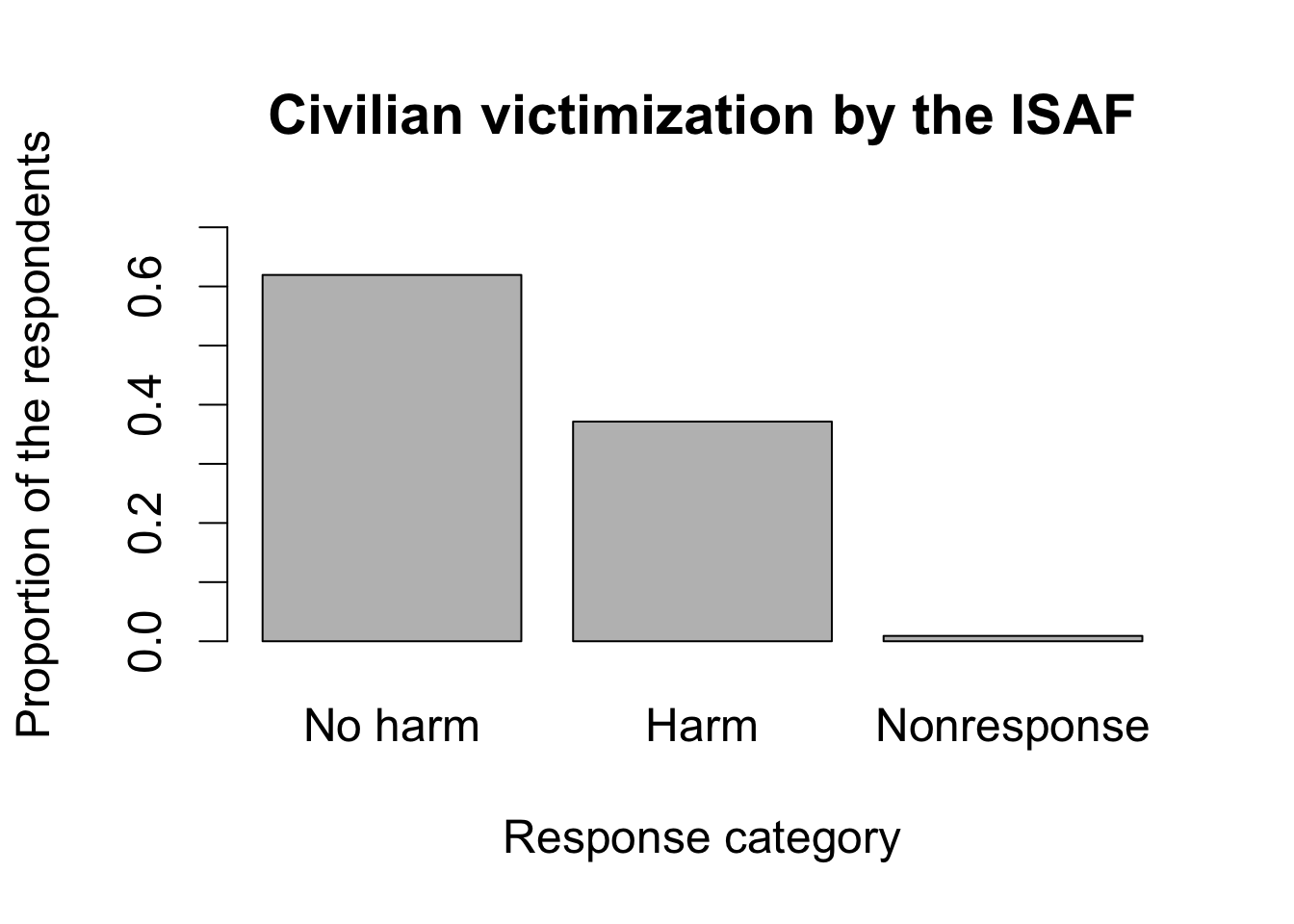## repeat the same for the victimization by Taliban
Taliban.ptable <- prop.table(table(Taliban = afghan$violent.exp.taliban, exclude = NULL)) barplot(Taliban.ptable, names.arg = c("No harm", "Harm", "Nonresponse"), main = "Civilian victimization by the Taliban", xlab = "Response category", ylab = "Proportion of the respondents", ylim = c(0, 0.7))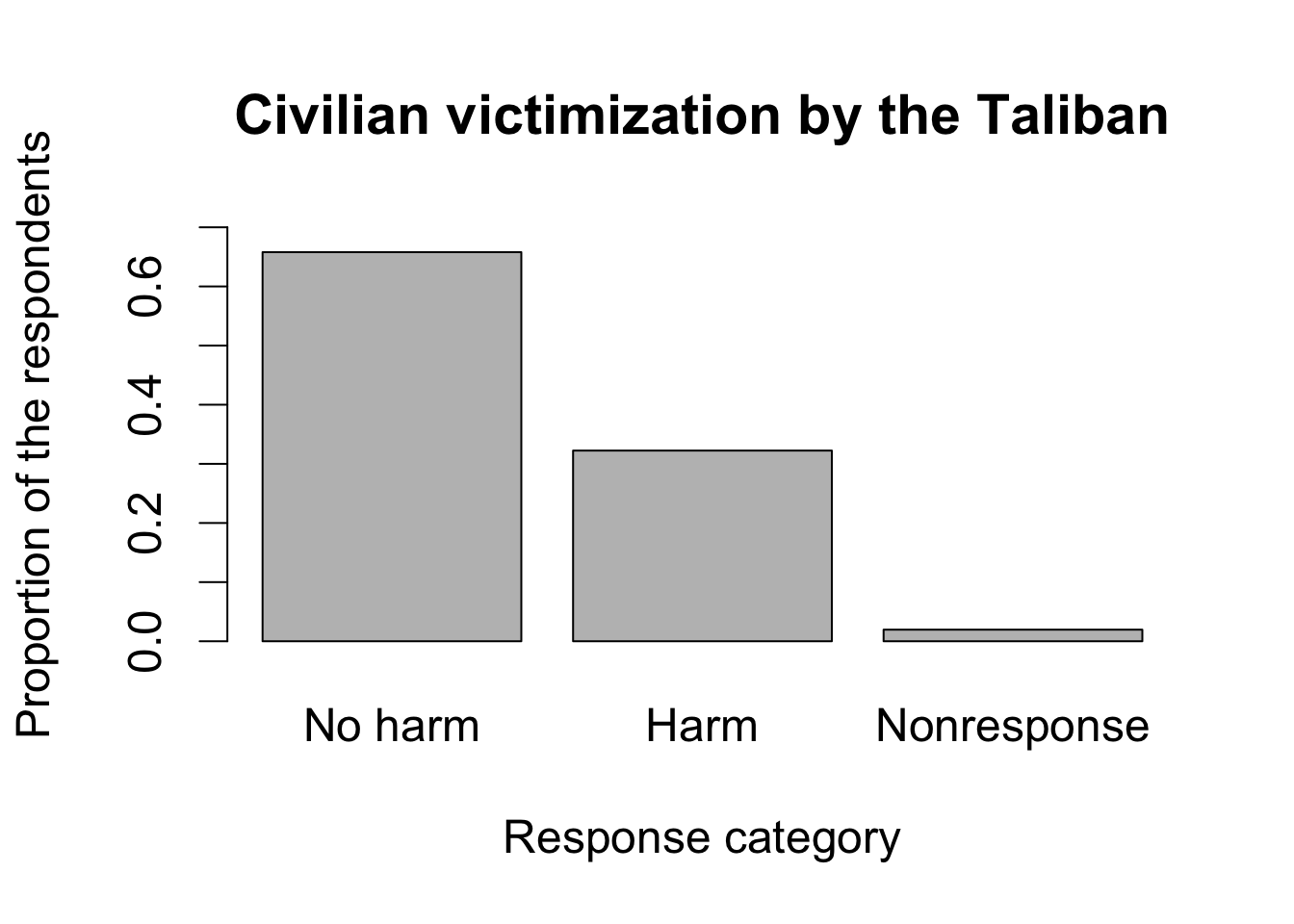### Section 3.3.2: Histogram par(cex = 1.5) hist(afghan$age, freq = FALSE, ylim = c(0, 0.04), xlab = "Age",
main = "Distribution of respondent's age")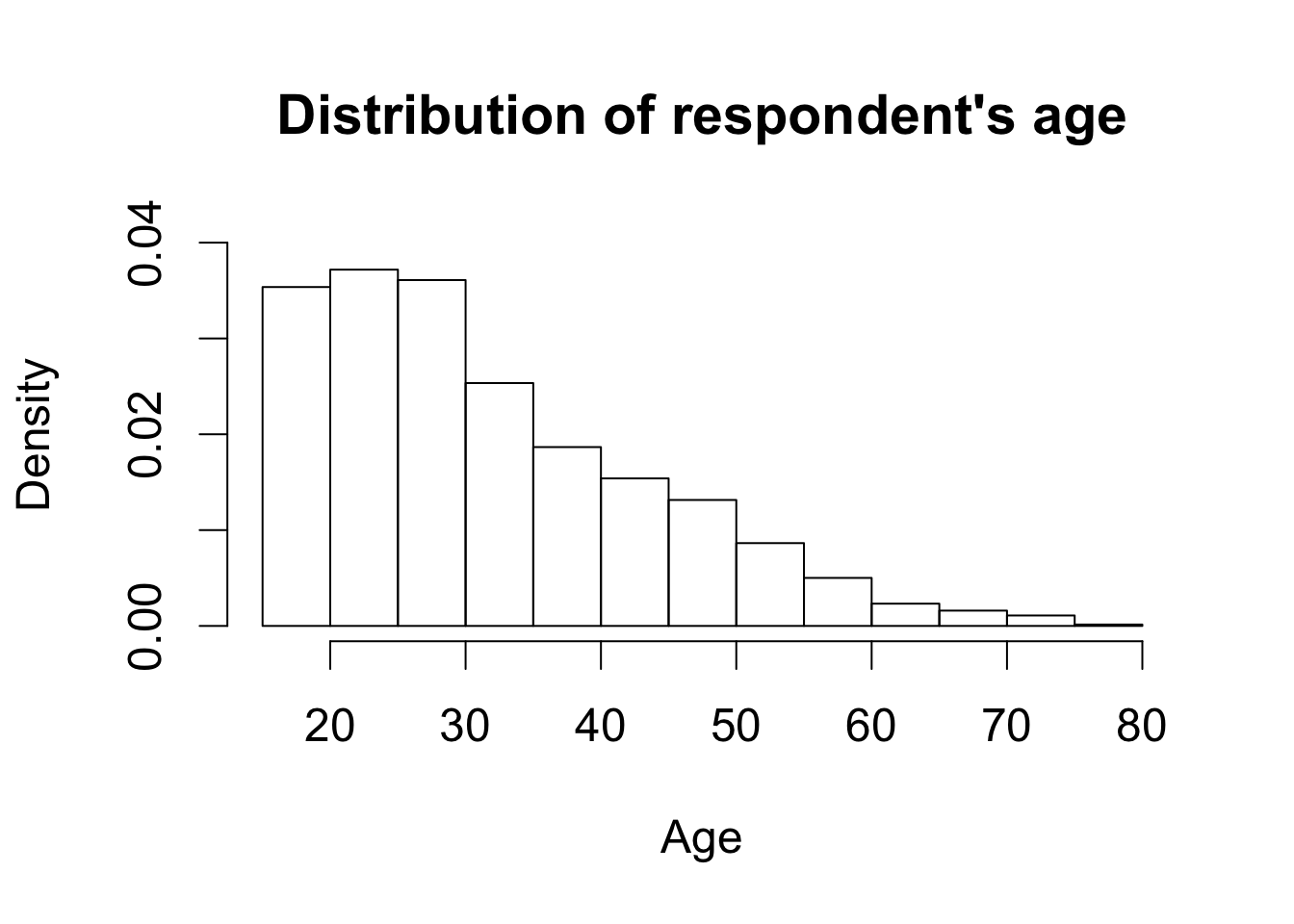par(cex = 1.5)
## histogram of education.  use breaks' to choose bins
hist(afghan$educ.years, freq = FALSE, breaks = seq(from = -0.5, to = 18.5, by = 1), xlab = "Years of education", main = "Distribution of respondent's education") ## add a text label at (x, y) = (3, 0.5) text(x = 3, y = 0.5, "median") ## add a vertical line representing median abline(v = median(afghan$educ.years))

## adding a vertical line representing median
lines(x = rep(median(afghan$educ.years), 2), y = c(0, 0.5))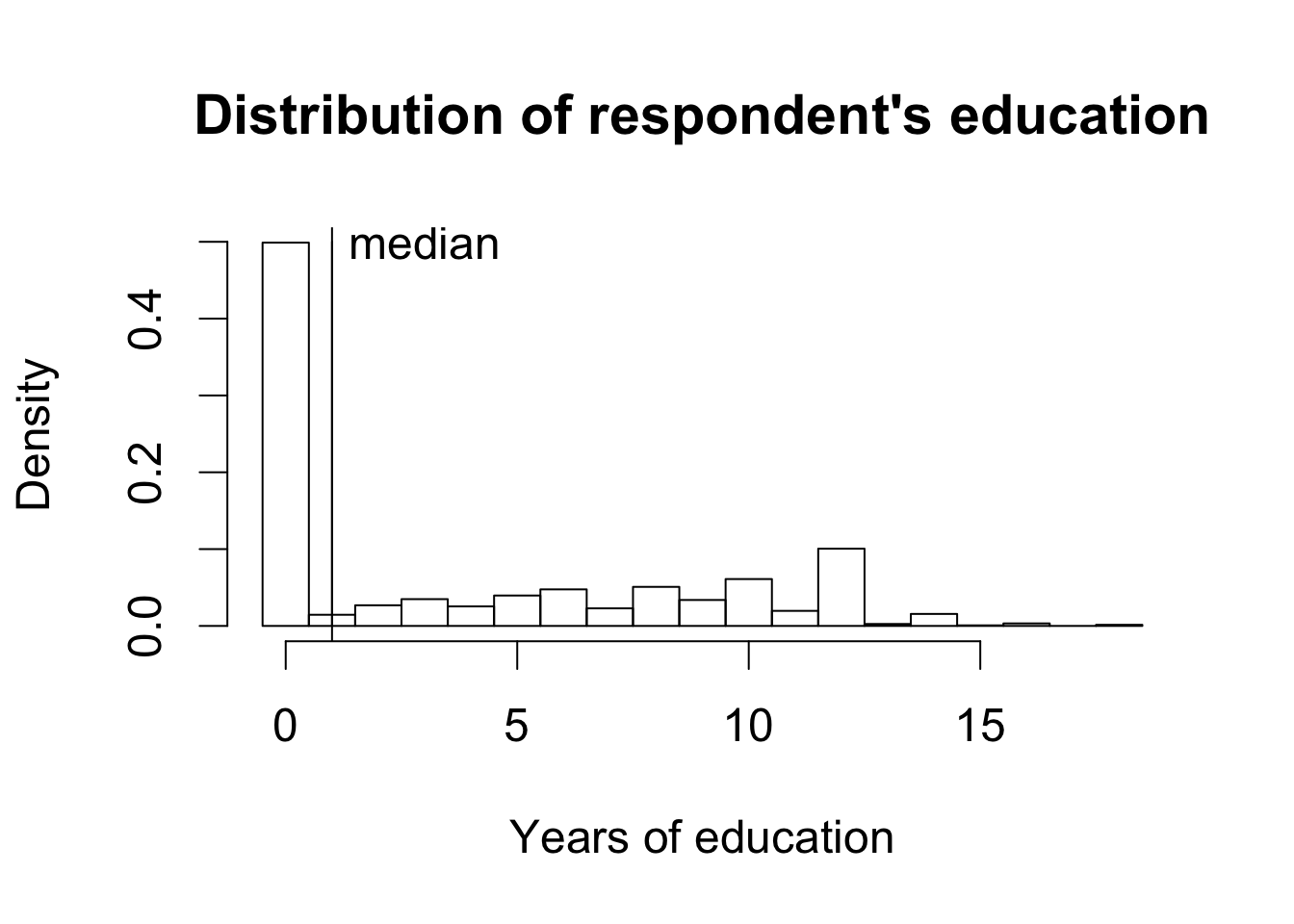### Section 3.3.3: Box Plot par(cex = 1.25) boxplot(educ.years ~ province, data = afghan, main = "Education by province", ylab = "Years of education")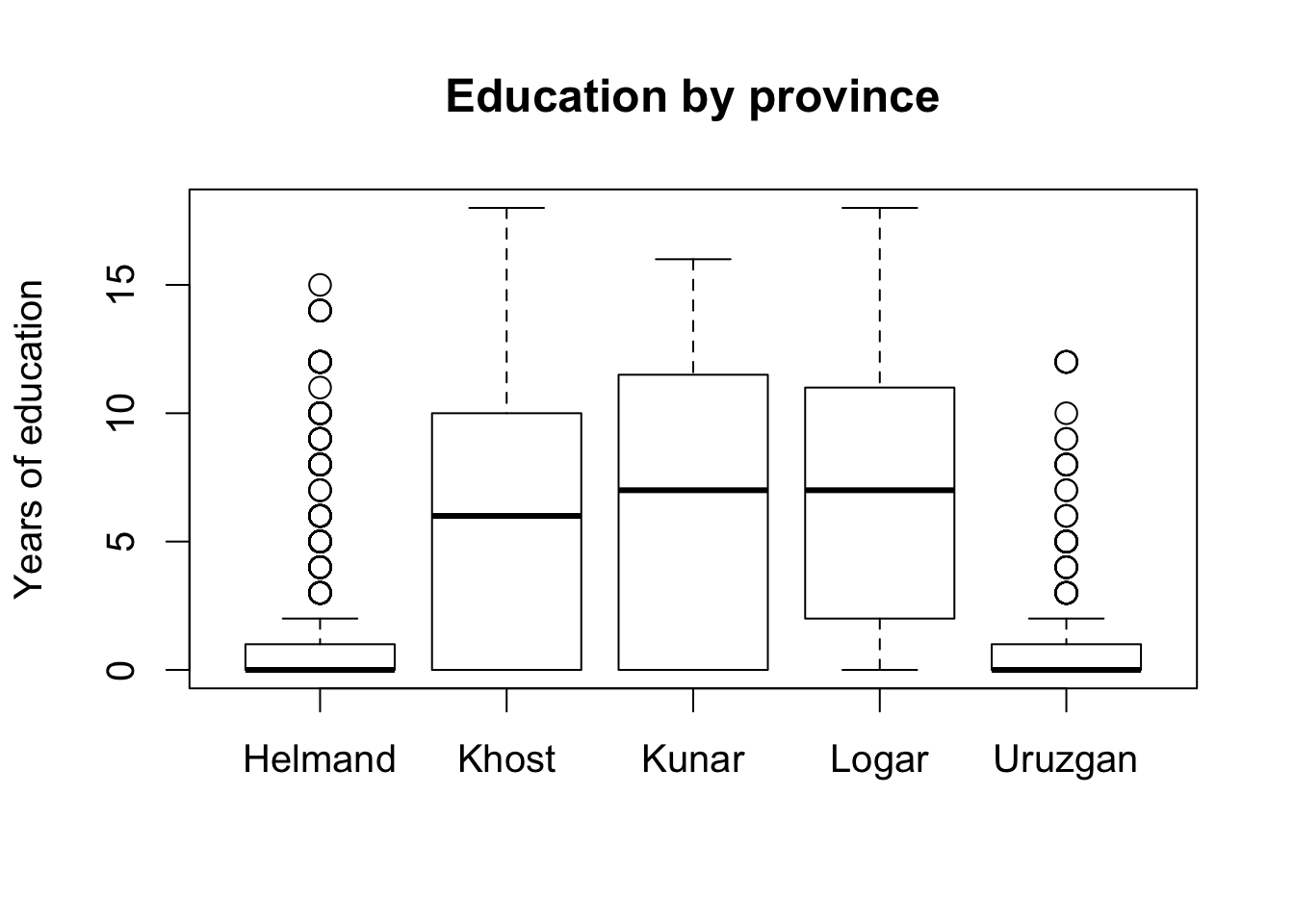tapply(afghan$violent.exp.taliban, afghan$province, mean, na.rm = TRUE) ## Helmand Khost Kunar Logar Uruzgan ## 0.50422195 0.23322684 0.30303030 0.08024691 0.45454545 tapply(afghan$violent.exp.ISAF, afghan$province, mean, na.rm = TRUE) ## Helmand Khost Kunar Logar Uruzgan ## 0.5410226 0.2424242 0.3989899 0.1440329 0.4960422 ## Saving or Printing a Graph ## pdf(file = "educ.pdf", height = 5, width = 5) ## boxplot(educ.years ~ province, data = afghan, ## main = "Education by Province", ylab = "Years of education") ## dev.off() ## pdf(file = "hist.pdf", height = 4, width = 8) ## ## one row with 2 plots with font size 0.8 ## par(mfrow = c(1, 2), cex = 0.8) ## ## for simplicity omit the texts and lines from the earlier example ## hist(afghan$age, freq = FALSE,
##      xlab = "Age", ylim = c(0, 0.04),
##      main = "Distribution of Respondent's Age")
## hist(afghan$educ.years, freq = FALSE, ## breaks = seq(from = -0.5, to = 18.5, by = 1), ## xlab = "Years of education", xlim = c(0, 20), ## main = "Distribution of Respondent's Education") ## dev.off() ## Section 3.4: Survey Sampling ### Section 3.4.1: The Role of Randomization par(cex = 1.5) ## load village data data("afghan.village", package = "qss") ## boxplots for altitude boxplot(altitude ~ village.surveyed, data = afghan.village, ylab = "Altitude (meter)", names = c("Nonsampled", "Sampled"))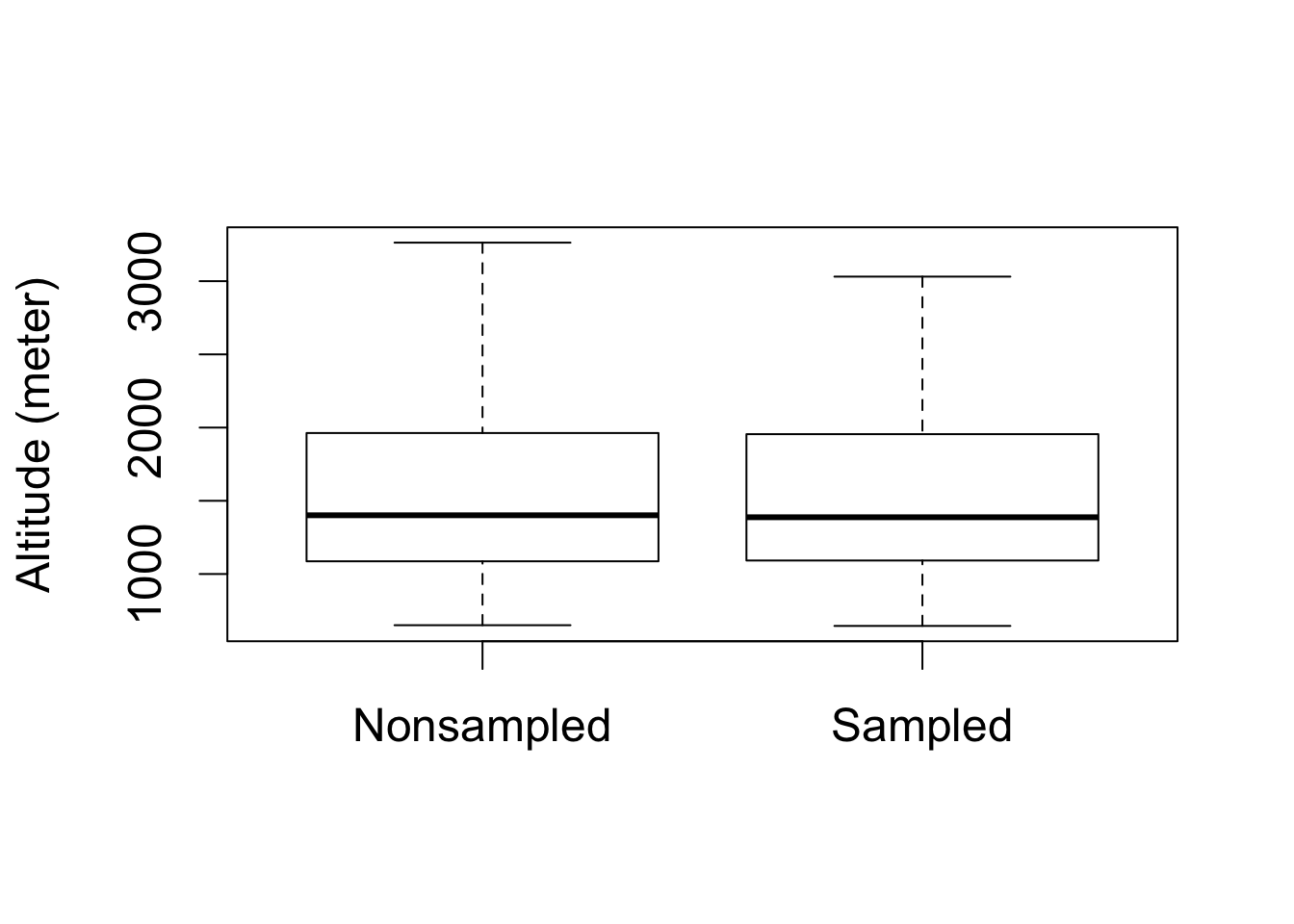## boxplots for log population boxplot(log(population) ~ village.surveyed, data = afghan.village, ylab = "log population", names = c("Nonsampled", "Sampled"))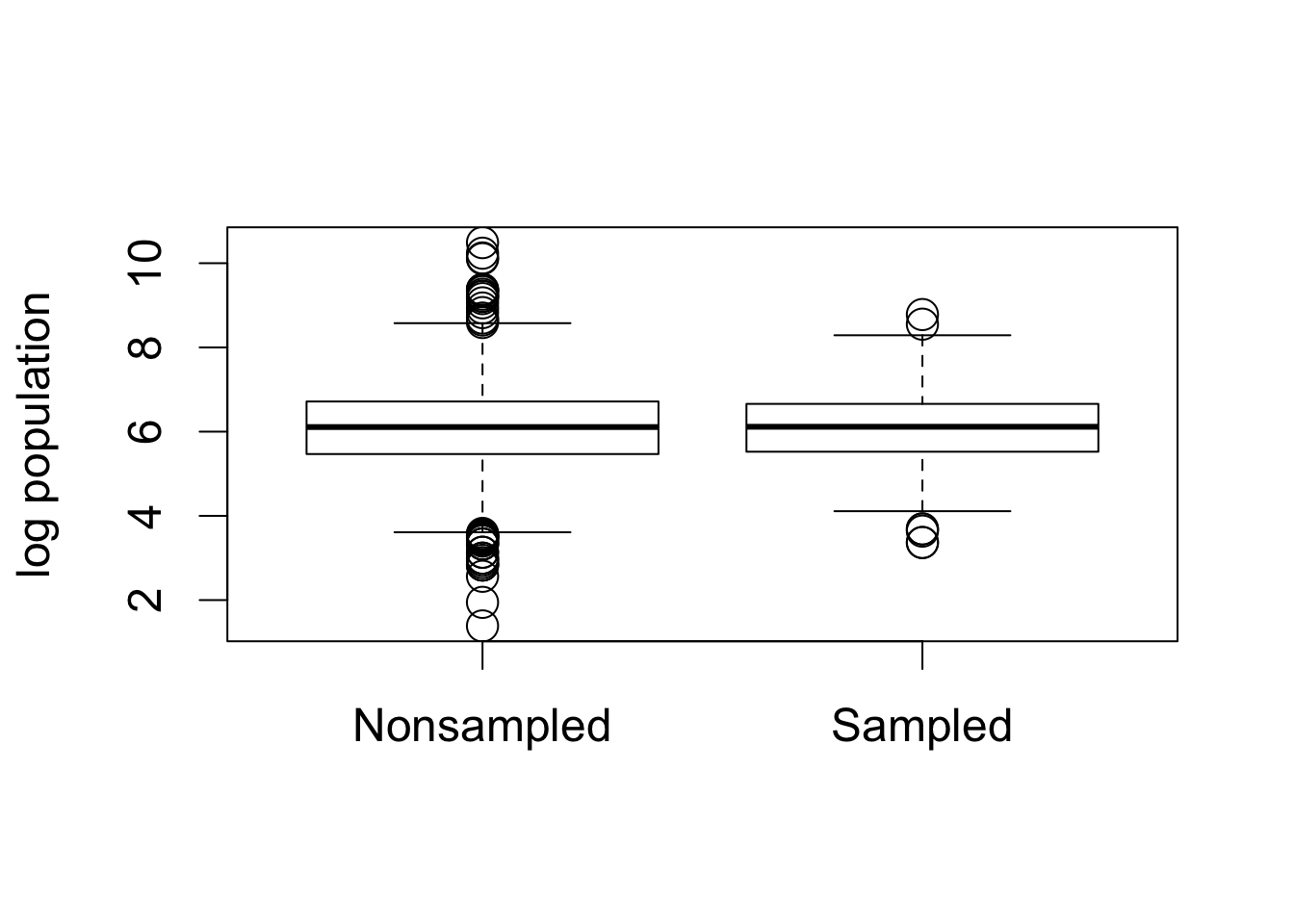### Section 3.4.2: Nonresponse and Other Sources of Bias tapply(is.na(afghan$violent.exp.taliban), afghan$province, mean) ## Helmand Khost Kunar Logar Uruzgan ## 0.030409357 0.006349206 0.000000000 0.000000000 0.062015504 tapply(is.na(afghan$violent.exp.ISAF), afghan$province, mean) ## Helmand Khost Kunar Logar Uruzgan ## 0.016374269 0.004761905 0.000000000 0.000000000 0.020671835 mean(afghan$list.response[afghan$list.group == "ISAF"]) - mean(afghan$list.response[afghan$list.group == "control"]) ##  0.04901961 table(response = afghan$list.response, group = afghan$list.group) ## group ## response control ISAF taliban ## 0 188 174 0 ## 1 265 278 433 ## 2 265 260 287 ## 3 200 182 198 ## 4 0 24 0 ## Section 3.5: Measuring Political Polarization ## Section 3.6: Summarizing Bivariate Relationships ### Section 3.6.1: Scatter Plot data("congress", package = "qss") ## subset the data by party rep <- subset(congress, subset = (party == "Republican")) dem <- congress[congress$party == "Democrat", ] # another way to subset

## 80th and 112th congress
rep80 <- subset(rep, subset = (congress == 80))
dem80 <- subset(dem, subset = (congress == 80))
rep112 <- subset(rep, subset = (congress == 112))
dem112 <- subset(dem, subset = (congress == 112))

## preparing the labels and axis limits to avoid repetition
xlab <- "Economic liberalism/conservatism"
ylab <- "Racial liberalism/conservatism"
lim <- c(-1.5, 1.5)

par(cex = 1.5)
## scatterplot for the 80th Congress
plot(dem80$dwnom1, dem80$dwnom2, pch = 16, col = "blue",
xlim = lim, ylim = lim, xlab = xlab, ylab = ylab,
main = "80th Congress") # democrats
points(rep80$dwnom1, rep80$dwnom2, pch = 17, col = "red") # republicans
text(-0.75, 1, "Democrats")
text(1, -1, "Republicans")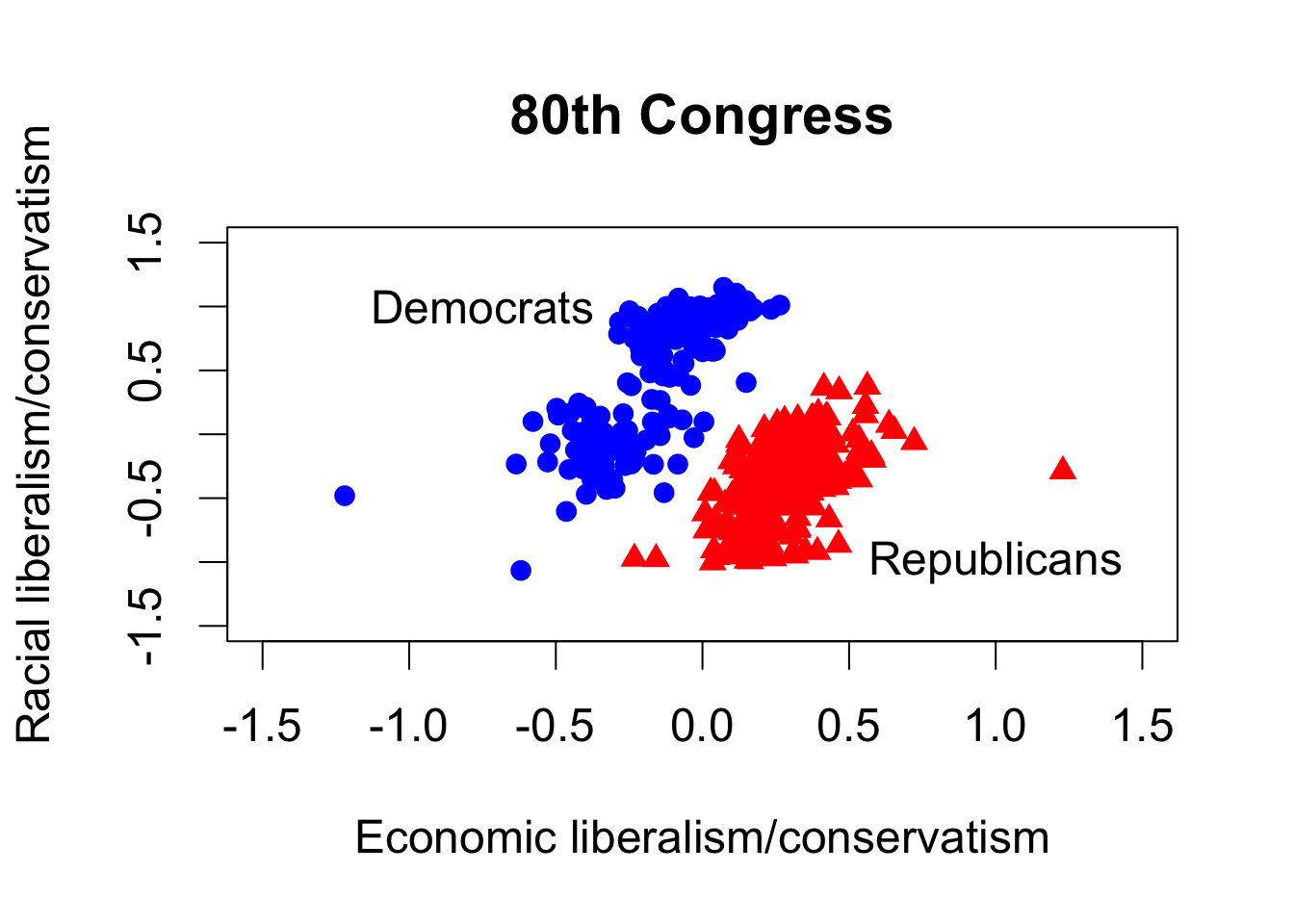## scatterplot for the 112th Congress
plot(dem112$dwnom1, dem112$dwnom2, pch = 16, col = "blue",
xlim = lim, ylim = lim, xlab = xlab, ylab = ylab,
main = "112th Congress")
points(rep112$dwnom1, rep112$dwnom2, pch = 17, col = "red")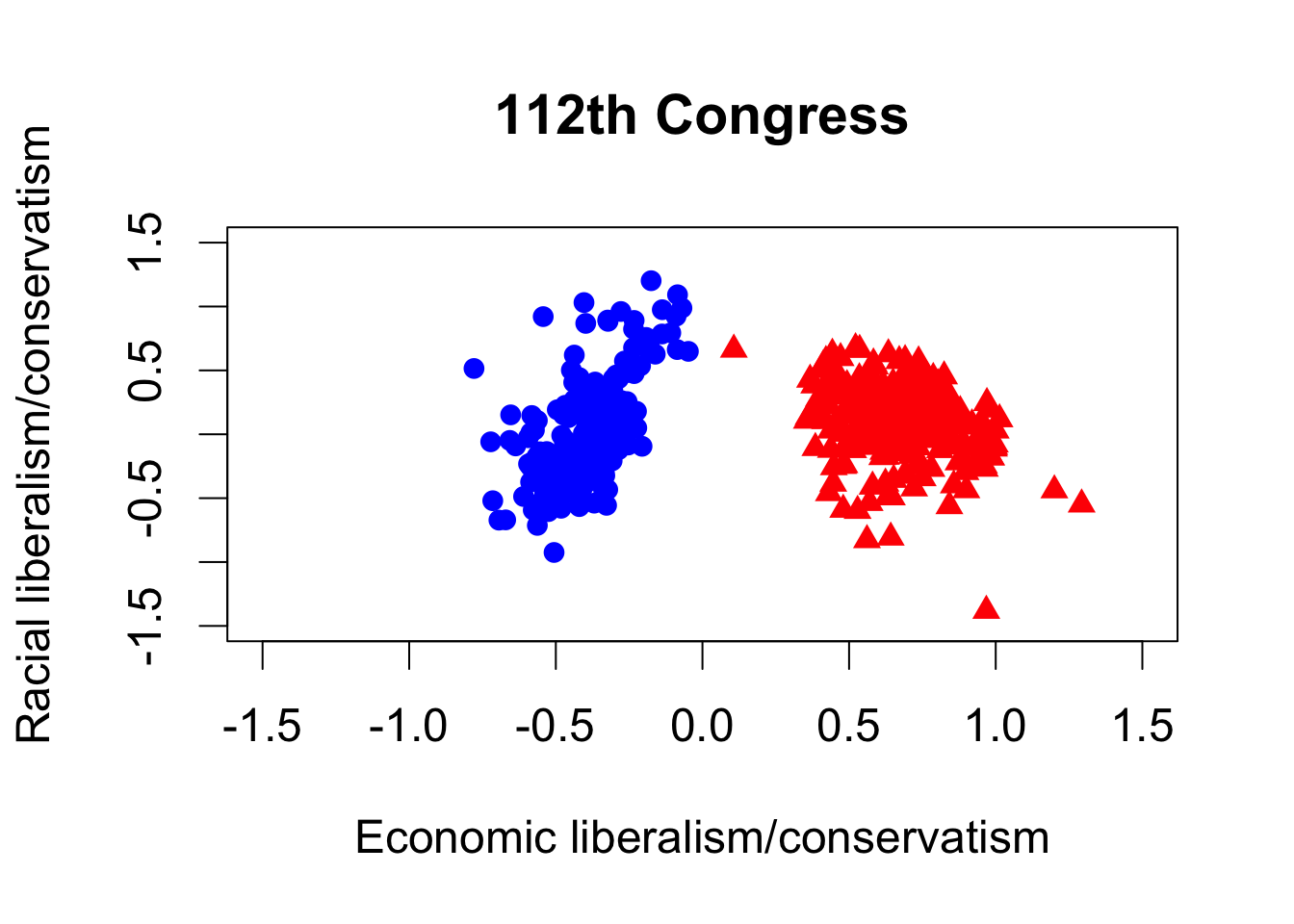## party median for each congress
dem.median <- tapply(dem$dwnom1, dem$congress, median)
rep.median <- tapply(rep$dwnom1, rep$congress, median)

par(cex = 1.5)
## Democrats
plot(names(dem.median), dem.median, col = "blue", type = "l",
xlim = c(80, 115), ylim = c(-1, 1), xlab = "Congress",
ylab = "DW-NOMINATE score (1st dimension)")
lines(names(rep.median), rep.median, col = "red")
text(110, -0.6, "Democratic\n Party")
text(110, 0.85, "Republican\n Party")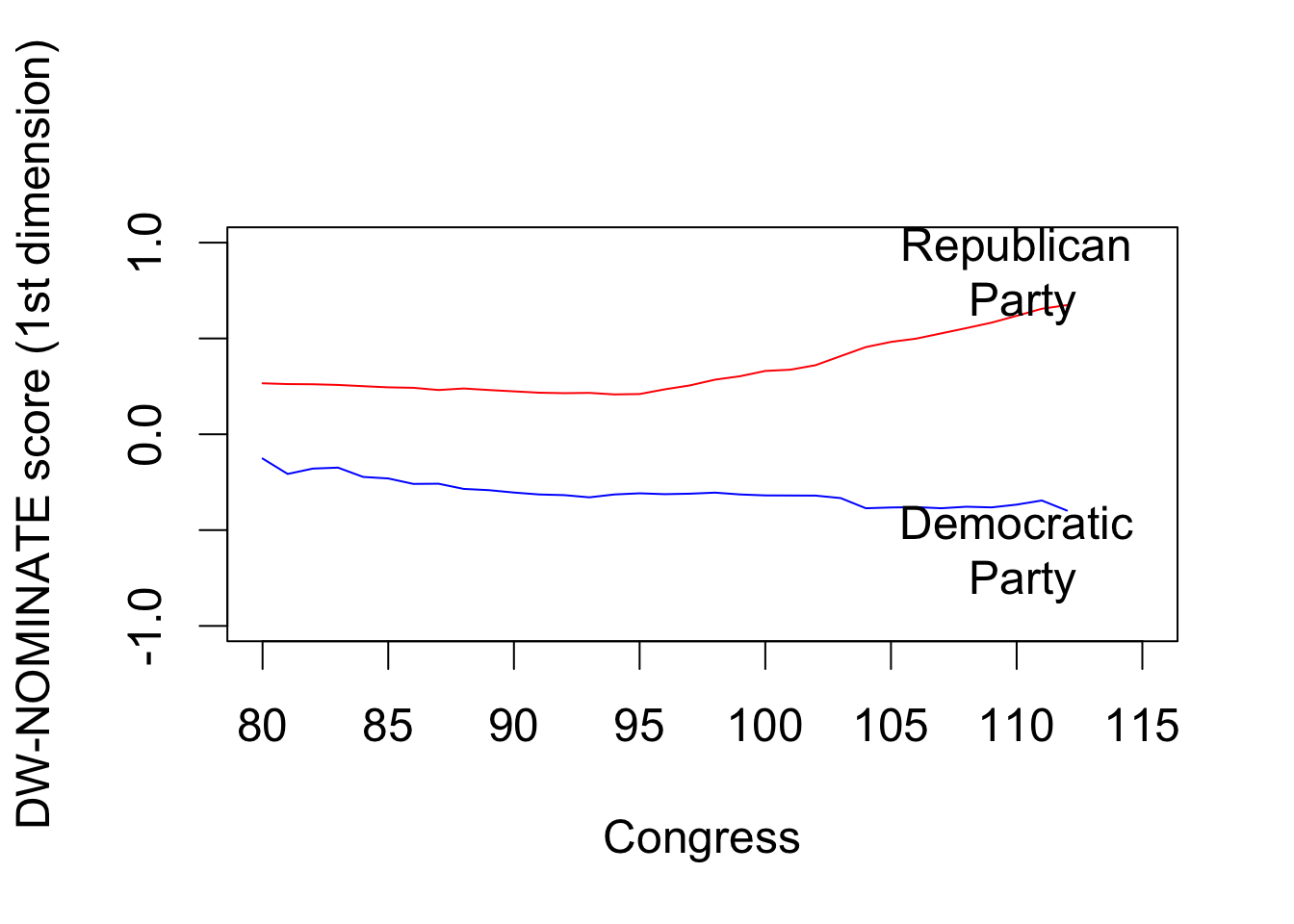### Section 3.6.2: Correlation

par(cex = 1.5)
## Gini coefficient data
data("USGini", package = "qss")

## time-series plot for partisan difference
plot(seq(from = 1947.5, to = 2011.5, by = 2),
rep.median - dem.median, xlab = "Year",
ylab = "Republican median -\n Democratic median",
main = "Political polarization")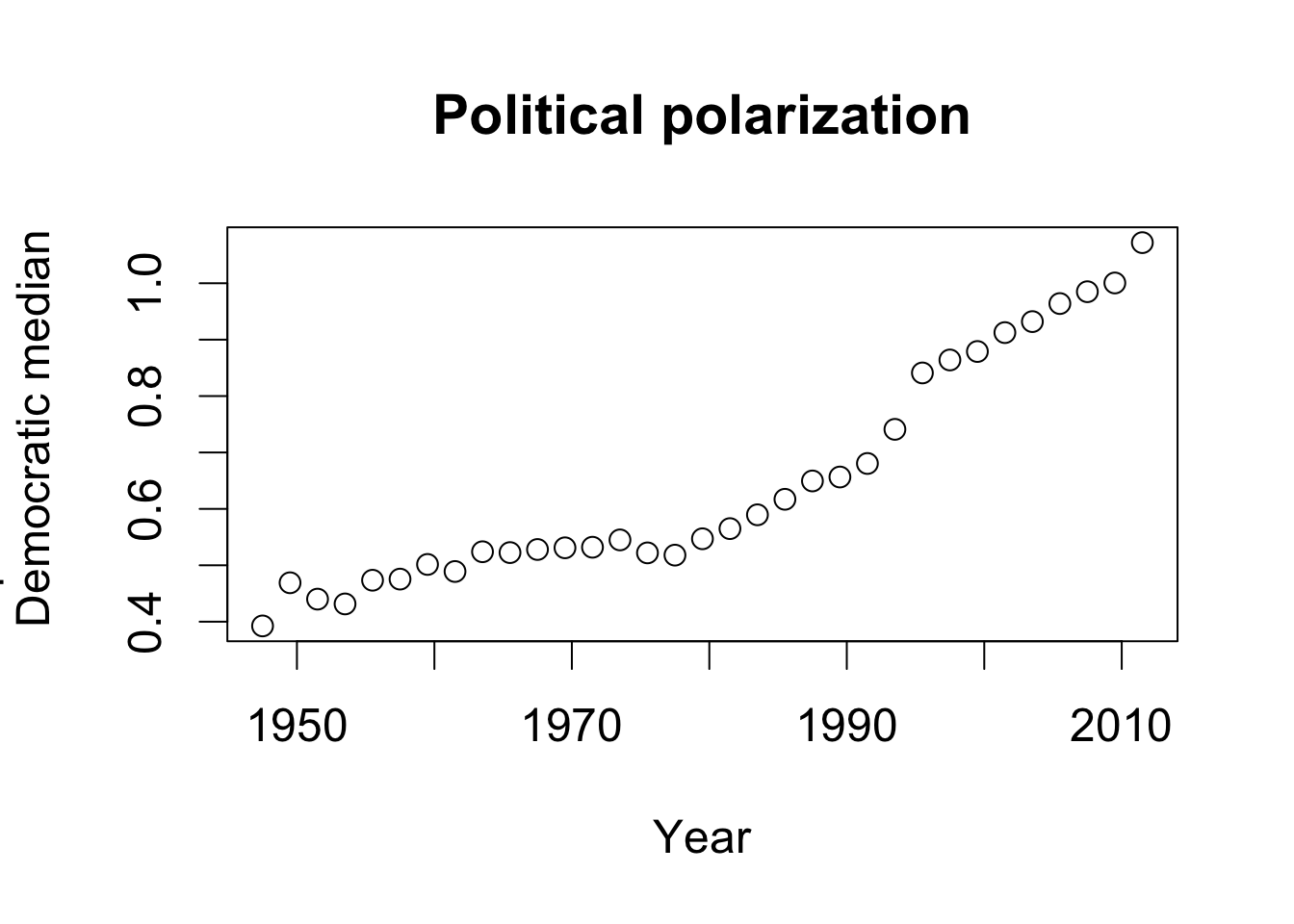## time-series plot for Gini coefficient
plot(USGini$year, USGini$gini, ylim = c(0.35, 0.45), xlab = "Year",
ylab = "Gini coefficient", main = "Income inequality")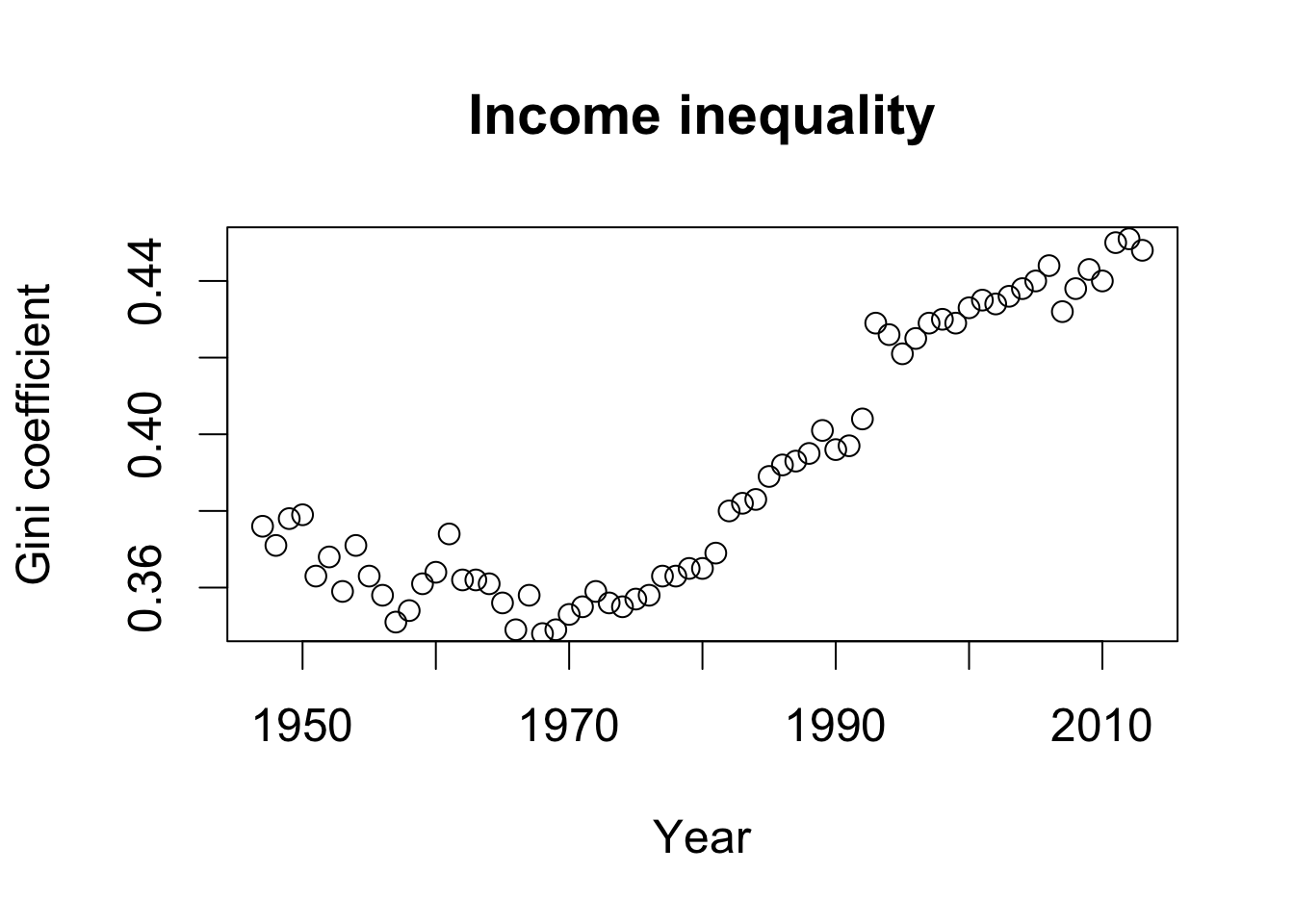cor(USGini$gini[seq(from = 2, to = nrow(USGini), by = 2)], rep.median - dem.median) ##  0.9418128 ### Section 3.6.3: Quantile-Quantile Plot par(cex = 1.5) hist(dem112$dwnom2, freq = FALSE, main = "Democrats",
xlim = c(-1.5, 1.5), ylim = c(0, 1.75),
xlab = "Racial liberalism/conservatism dimension")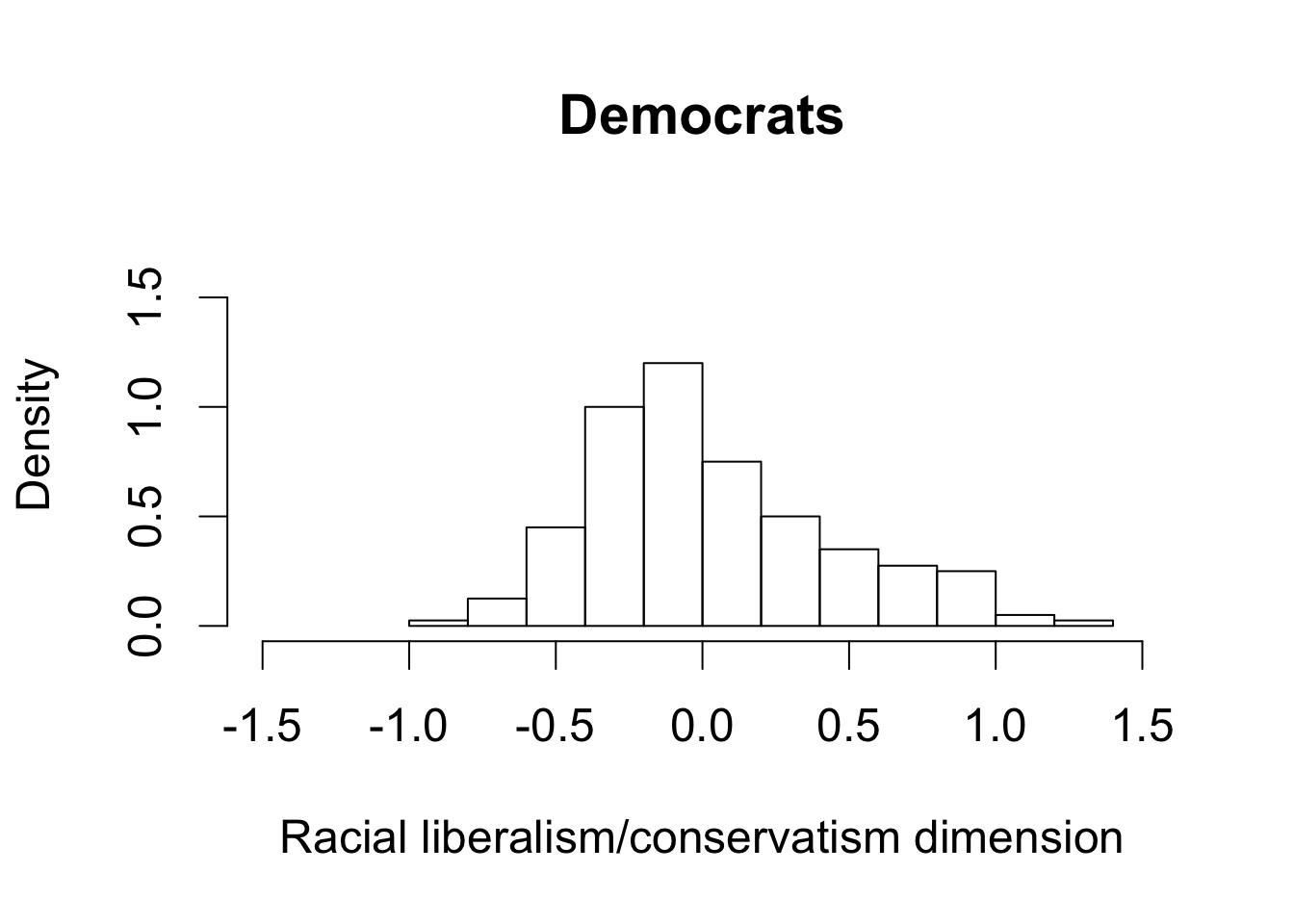hist(rep112$dwnom2, freq = FALSE, main = "Republicans", xlim = c(-1.5, 1.5), ylim = c(0, 1.75), xlab = "Racial liberalism/conservatism dimension")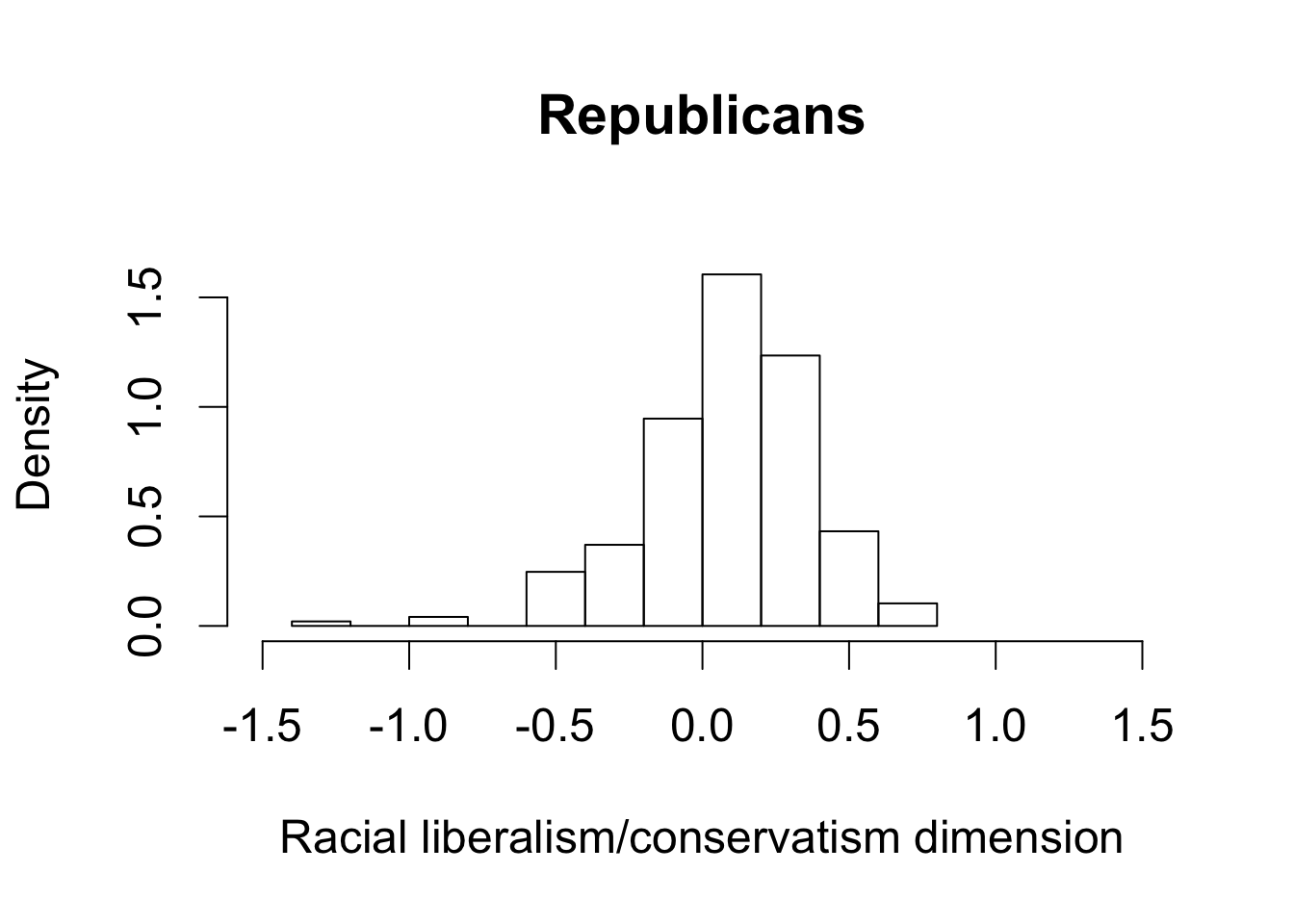par(cex = 1.5) qqplot(dem112$dwnom2, rep112$dwnom2, xlab = "Democrats", ylab = "Republicans", xlim = c(-1.5, 1.5), ylim = c(-1.5, 1.5), main = "Racial liberalism/conservatism dimension") abline(0, 1) # 45 degree line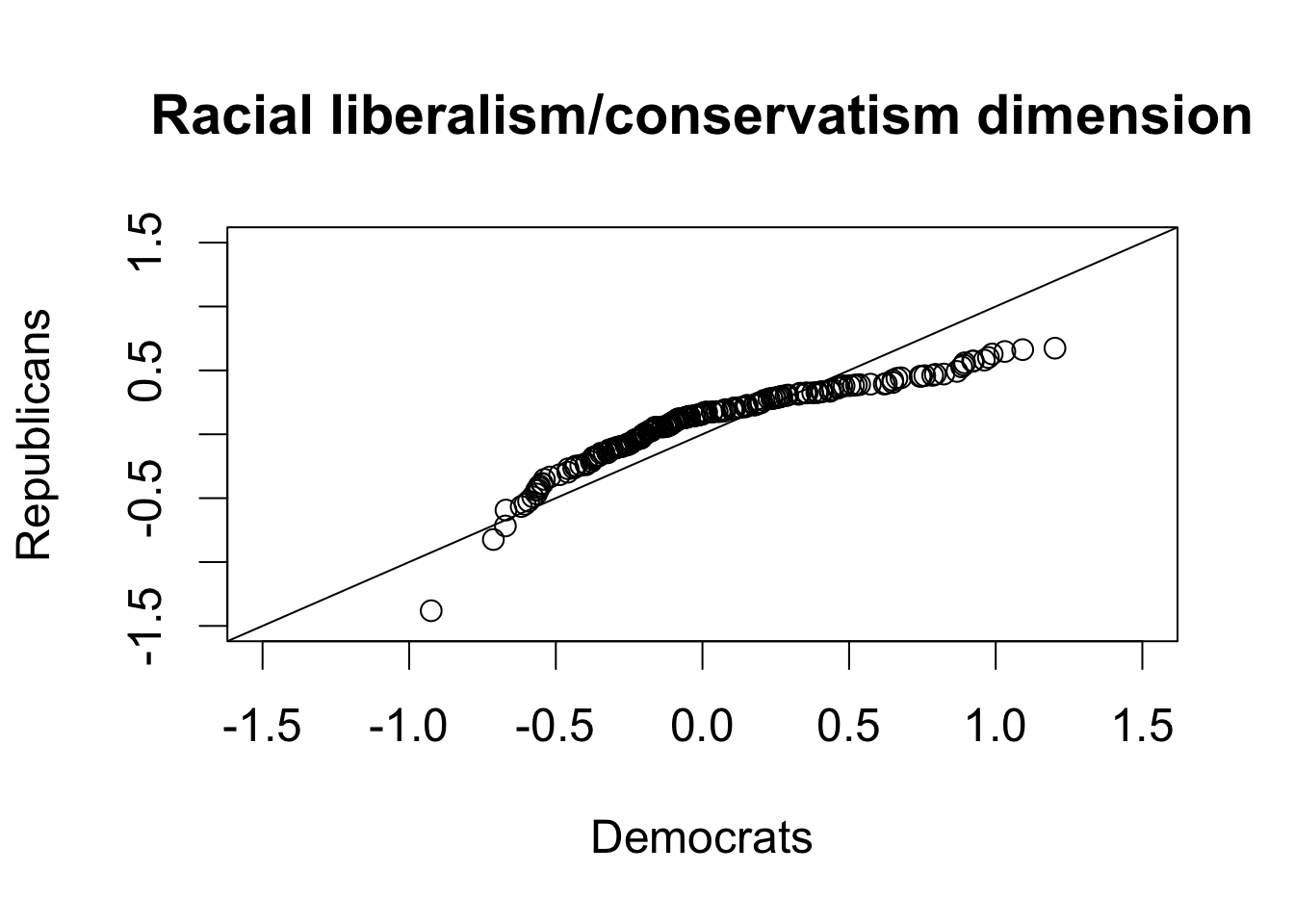## Section 3.7: Clustering ## 3x4 matrix filled by row; first argument take actual entries x <- matrix(1:12, nrow = 3, ncol = 4, byrow = TRUE) rownames(x) <- c("a", "b", "c") colnames(x) <- c("d", "e", "f", "g") dim(x) # dimension ##  3 4 x ## d e f g ## a 1 2 3 4 ## b 5 6 7 8 ## c 9 10 11 12 ## data frame can take different data types y <- data.frame(y1 = as.factor(c("a", "b", "c")), y2 = c(0.1, 0.2, 0.3)) class(y$y1)
##  "factor"
class(y$y2) ##  "numeric" ## as.matrix() converts both variables to character z <- as.matrix(y) z ## y1 y2 ## [1,] "a" "0.1" ## [2,] "b" "0.2" ## [3,] "c" "0.3" ## column sums colSums(x) ## d e f g ## 15 18 21 24 ## row means rowMeans(x) ## a b c ## 2.5 6.5 10.5 ## column sums apply(x, 2, sum) ## d e f g ## 15 18 21 24 ## row means apply(x, 1, mean) ## a b c ## 2.5 6.5 10.5 ## standard deviation for each row apply(x, 1, sd) ## a b c ## 1.290994 1.290994 1.290994 ### Section 3.7.2: List in R ## create a list x <- list(y1 = 1:10, y2 = c("hi", "hello", "hey"), y3 = data.frame(z1 = 1:3, z2 = c("good", "bad", "ugly"))) ## 3 ways of extracting elements from a list x$y1 # first element
##    1  2  3  4  5  6  7  8  9 10
x[] # second element
##  "hi"    "hello" "hey"
x[["y3"]] # third element
##   z1   z2
## 1  1 good
## 3  3 ugly

### Section 3.7.3: The k-Means Algorithm

names(x)  # names of all elements
##  "y1" "y2" "y3"
length(x) # number of elements
##  3
dwnom80 <- cbind(congress$dwnom1[congress$congress == 80],
congress$dwnom2[congress$congress == 80])
dwnom112 <- cbind(congress$dwnom1[congress$congress == 112],
congress$dwnom2[congress$congress == 112])

## kmeans with 2 clusters
k80two.out <- kmeans(dwnom80, centers = 2, nstart = 5)
k112two.out <- kmeans(dwnom112, centers = 2, nstart = 5)

## elements of a list
names(k80two.out)
##  "cluster"      "centers"      "totss"        "withinss"
##  "tot.withinss" "betweenss"    "size"         "iter"
##  "ifault"
## final centroids
k80two.out$centers ## [,1] [,2] ## 1 0.14681029 -0.3389293 ## 2 -0.04843704 0.7827259 k112two.out$centers
##         [,1]       [,2]
## 1 -0.3912687 0.03260696
## 2  0.6776736 0.09061157
## number of observations for each cluster by party
table(party = congress$party[congress$congress == 80],
cluster = k80two.out$cluster) ## cluster ## party 1 2 ## Democrat 62 132 ## Other 2 0 ## Republican 247 3 table(party = congress$party[congress$congress == 112], cluster = k112two.out$cluster)
##             cluster
## party          1   2
##   Democrat   200   0
##   Republican   1 242
## kmeans with 4 clusters
k80four.out <- kmeans(dwnom80, centers = 4, nstart = 5)
k112four.out <- kmeans(dwnom112, centers = 4, nstart = 5)

par(cex = 1.5)
## plotting the results using the labels and limits defined earlier
plot(dwnom80, col = k80four.out$cluster + 1, xlab = xlab, ylab = ylab, xlim = lim, ylim = lim, main = "80th Congress") ## plotting the centroids points(k80four.out$centers, pch = 8, cex = 2)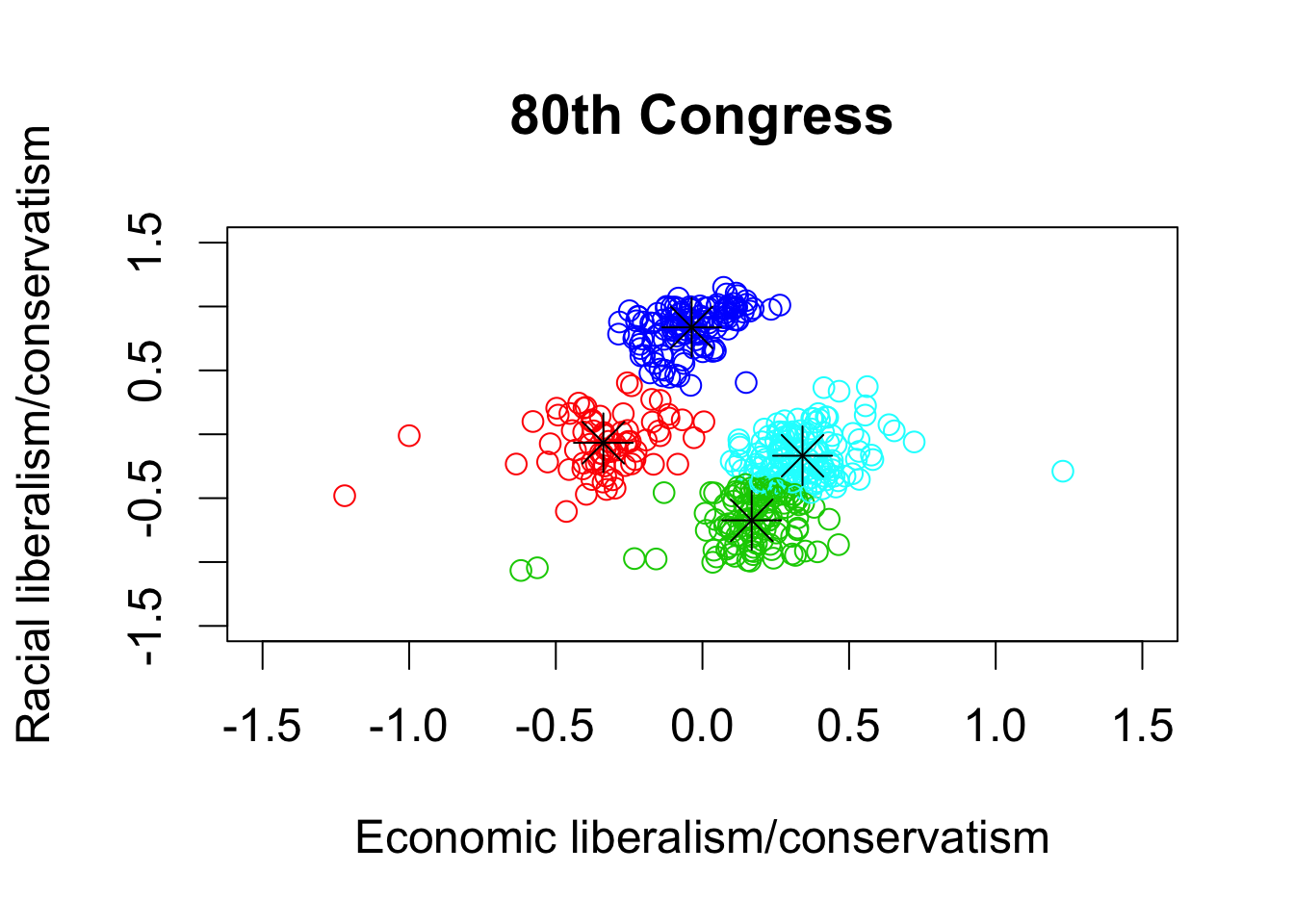## 112th congress
plot(dwnom112, col = k112four.out$cluster + 1, xlab = xlab, ylab = ylab, xlim = lim, ylim = lim, main = "112th Congress") points(k112four.out$centers, pch = 8, cex = 2)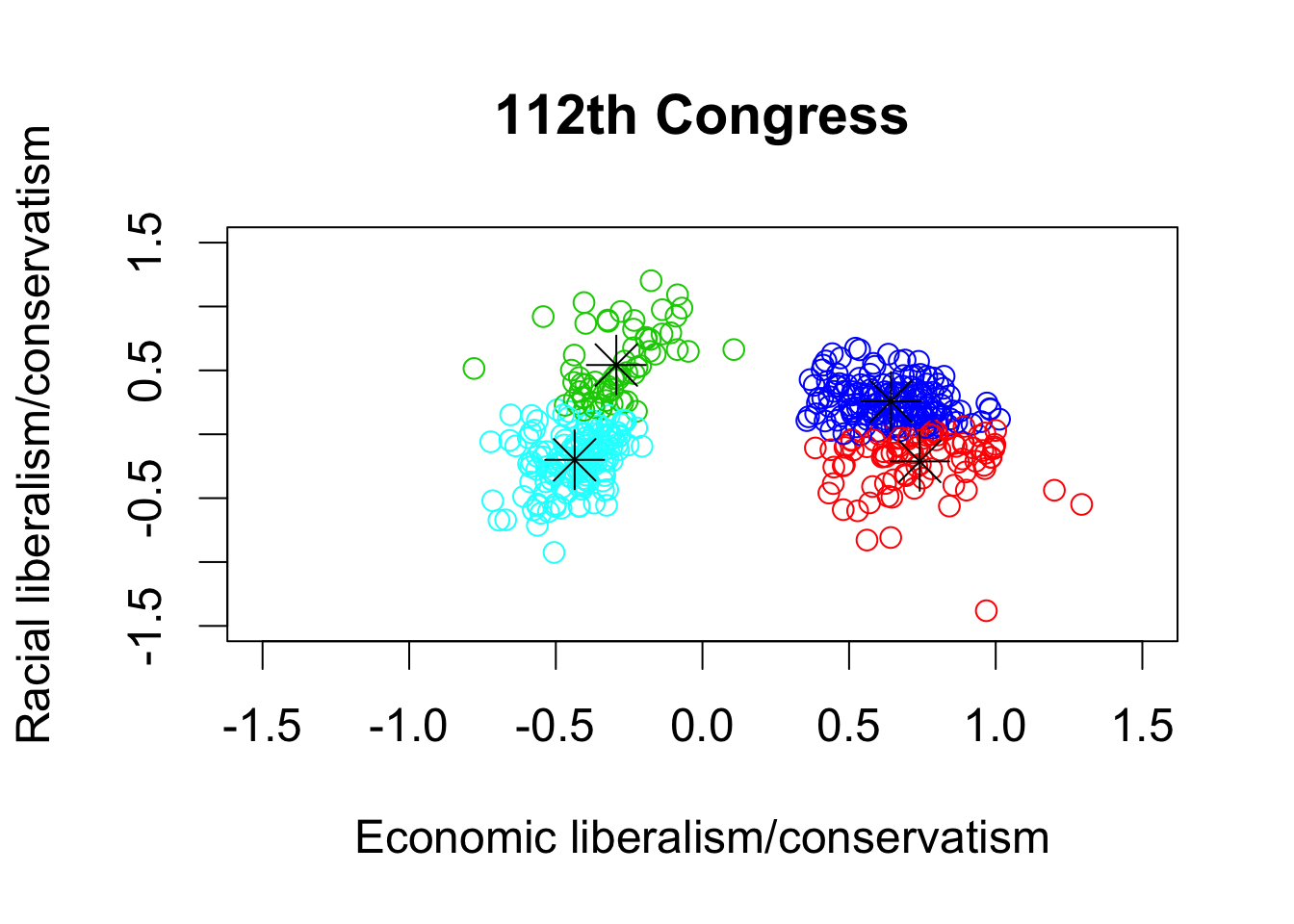palette()
##  "black"   "red"     "green3"  "blue"    "cyan"    "magenta" "yellow"
##  "gray"`